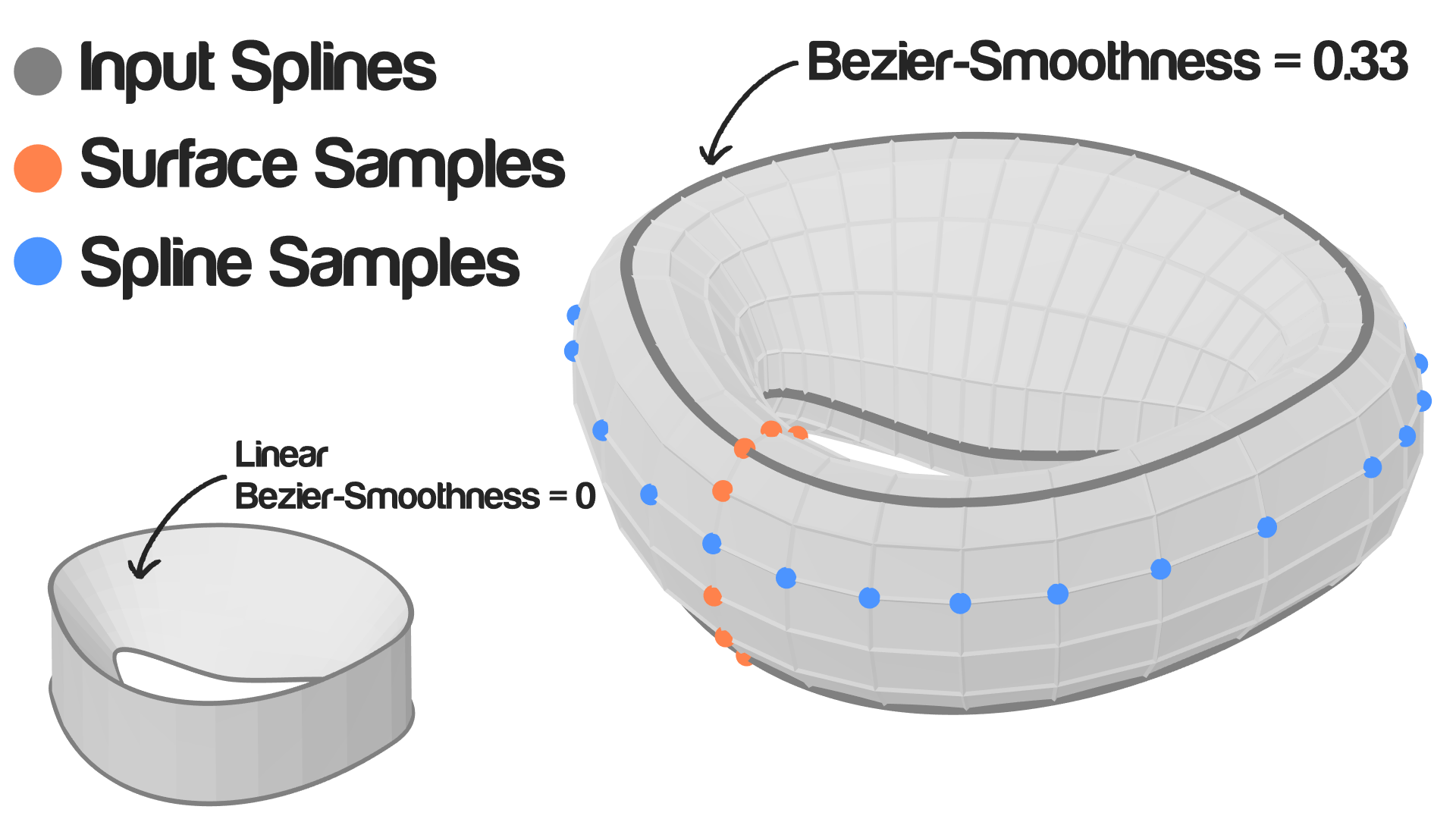# Loft Splines

## Description

This node generates a mesh by sampling some points on the input splines and bridge (connect) them in order.

## Options

• Bezier - This option will use a bezier interpolation to locate the segments of the bridge between the splines and thus having a smooth output mesh.
• Linear - This option will use a linear interpolation to locate the segments of the bridge between the splines and thus having a flat connection between the splines.

If the Surface Samples is set to 2 or the Smoothness is set to zero, the bezier interpolation will work just as a linear interpolation.

## Illustration## Inputs

• Splines - A list of splines to be connected.
• Spline Samples - The amount of vertices sampled and used to create the mesh.
• Surface Samples - The amount of segments created between the splines.
• Cyclic - A boolean which if true will connect the polygons vertically and thus having a manifold object.
• Smoothness - A factor that define the smoothness of the beizer interpolation.(Only when using Bezier option)
• Start - The normalized distance at which the first loop will start.
• End - The normalized distance at which the first loop will end.

## Outputs

• Vertices - A vector list that contains the vertices locations of the output mesh.
• Polygons - A polygon indices list that carry the polygons information of the output mesh.